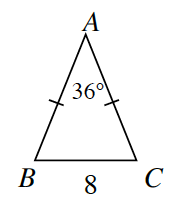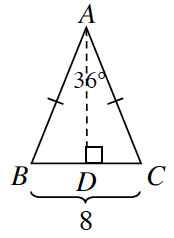### Home > CCG > Chapter Ch7 > Lesson 7.2.3 > Problem7-78

7-78.

Tromika wants to find the area of the isosceles triangle below.1.She decided to start by drawing a height from vertex $A$ to side $\overline{BC}$ as shown at right. Will the two smaller triangles be congruent? In other words, is $ΔABD≅ΔACD$? Why or why not?

Look at the two triangles that are created. They share a side, and the tick marks mean the side lengths are equal. Are the triangles congruent?

Yes, they are congruent by $\text{HL}≅$.

2. What is $m∠DAB$? $BD$?

$∠DAB≅∠DAC$ since congruent triangles have congruent parts.

$m∠DAB=18°$
$BD=4$

3. Find $AD$. Show how you got your answer.

Which trig ratio can you use to determine this length?

4. Find the area of $ΔABC$.

How do you find the area of a triangle? Do you have enough information to calculate the area?